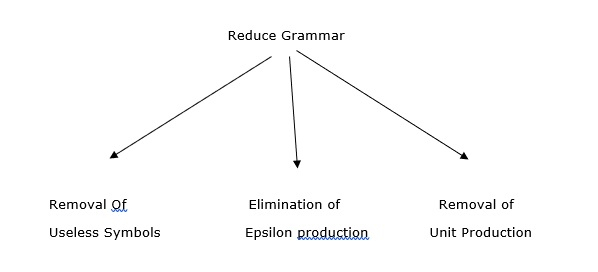# Explain the simplification of context free grammar in TOC

A context free grammar (CFG) is a forma grammar which is used to generate all possible patterns of strings in a given formal language.

It is defined as four tuples −

G=(V,T,P,S)

Where,

• G is a grammar, which consists of a set of production rules. It is used to generate the strings of a language.
• T is the final set of terminal symbols. It is denoted by lower case letters.
• V is the final set of non-terminal symbols. It is denoted by capital letters
• P is a set of production rules, which is used for replacing non-terminal symbols (on the left side of production) in a string with other terminals (on the right side of production).
• S is the start symbol used to derive the string

All grammars are not always optimized, which means the grammar may consist of some extra symbols(non-terminals) which increase the length of grammar.

So, we have to reduce the grammar by removing useless symbols.

## Properties

The properties to reduce the grammar are as follows −

• Each non-terminal and terminal of G appears in the derivation of some word in L.
• There should not be any production as X->Y where X and Y are non-terminals.
• If epsilon is not in language L then there need not be in the production X-> ε.

The uses of reducing the grammar are explained below −